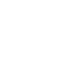## EXPERTISE###### QUANTITATIVE FINANCE

Modeling and Pricing

• Interest Rates, FX/Equity and Inflation

• Pricing and hedging under multi-curve framework

• Interest Rates volatility cube construction

• Volatility surface calibration

• Yield Curve bootstrapping

Risk Management

• Value at Risk calculation: Monte Carlo, Historical and parametric

• Initial Margin calculation and backtesting

• Multi-objective constrained portfolio optimization

• Monte Carlo simulation models###### DATA SCIENCE

Machine Learning

• Supervised Learning, Linear Models,      Support Vector Machine, Decision Trees

• Assembled Models

• Unsupervised Learning

NLP

• Text processing

• Article summarization

• Entity extraction

• Word embeddings

Deep Learning

• Convolutional Neural Networks

• Recurrent Neural Networks

• Generative Adversarial Networks

• Deep Reinforcement Learning

###### RESERVOIR ENGINEERING

Field Development

• Optimization of production and reserves

• Identification of best drilling targets

• Optimization of drilling/workovers

• Evaluation of secondary/tertiary recovery methods (EOR screening)

• Artificial Lift Optimization/ Well Testing

• Reservoir Simulation

Portfolio Management

• Engineering and Economic asset valuation

• Multi-objective Optimization of Portfolio

• Diagnostic of Oil Field potential value

• Optimization

• Uncertainty and risk quantification

• Data driven methods for portfolio optimization